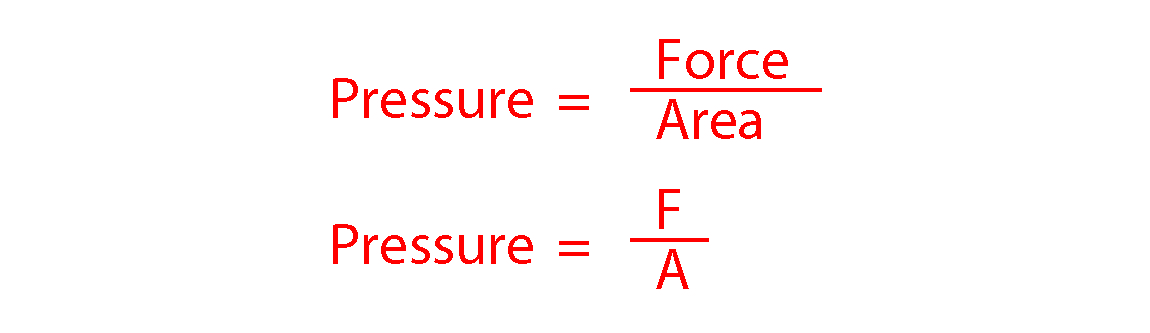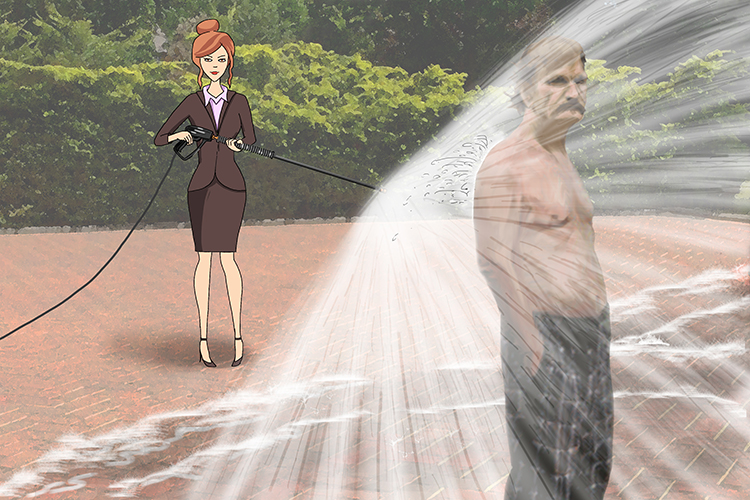# Pressure

Pressure is a continual force applied on or against an object's surface by something in contact with it. The formula for pressure is given below:The pressure washer forced water over a wide area.

NOTE:

Various units are used for pressure. The ones used in common everyday language include bar, pounds per square inch (psi) and standard atmospheric pressure (atm). However the standard unit you need to know and will most likely have to use in the exam is pascal (Pa) which is equal to 1 Newton per square metre. This unit of measurement was named after a Frenchman called Blaise Pascal. Pascals are small units, so kilopascals (1000 pascals, written kPa) are often used.

To remember that pressure is measured in pascals think of this picture:The personal assistant (Pa) sprayed her Pa (Pa) with the pressure washer because he accidentally got in the way.

Example 1

Figure 17 shows how atmospheric pressure varies with altitude.When flying, the pressure inside the cabin of an aircraft is kept at 70 kPa. The aircraft window has an area of 810 cm^2. Use data from Figure 17 to calculate the resultant force acting on an aircraft window when the aircraft is flying at an altitude of 12 km. Give your answer to two significant figures.From the graph we can calculate the atmospheric pressure outside the window of the aircraft to be 20kPa.

The area of the window = 810 cm2 = 0.081 m2

Net pressure = 70 kPa – 20 kPa = 50 kPa = 50000 Pa

pressure=\frac{Force}{area}

Multiply both sides by area to make force the subject:

pressure\times area=\ \frac{Force}{\class{strike}{area}}\times \class{strike}{area}

Force = pressure x area

Therefore the resultant Force = 50000 x 0.081 = 4050N.

Correct to two significant figures the resultant Force = 4100N.